# RS Aggarwal Solutions for Class 12 Maths Chapter 11: Applications of Derivatives Exercise 11H

Tangents and Normals along with solved examples are the topics discussed in brief under the Exercise 11H of Chapter 11. This exercise also explains important theorems which are important from the exam point of view. The solutions are prepared in an interactive manner to make it interesting for students during the exam preparation. To score well in the board exam, students can download RS Aggarwal Solutions Class 12 Maths Chapter 11 Applications of Derivatives Exercise 11H PDF with the help of links which are given here.

## RS Aggarwal Solutions for Class 12 Maths Chapter 11: Applications of Derivatives Exercise 11H Download PDF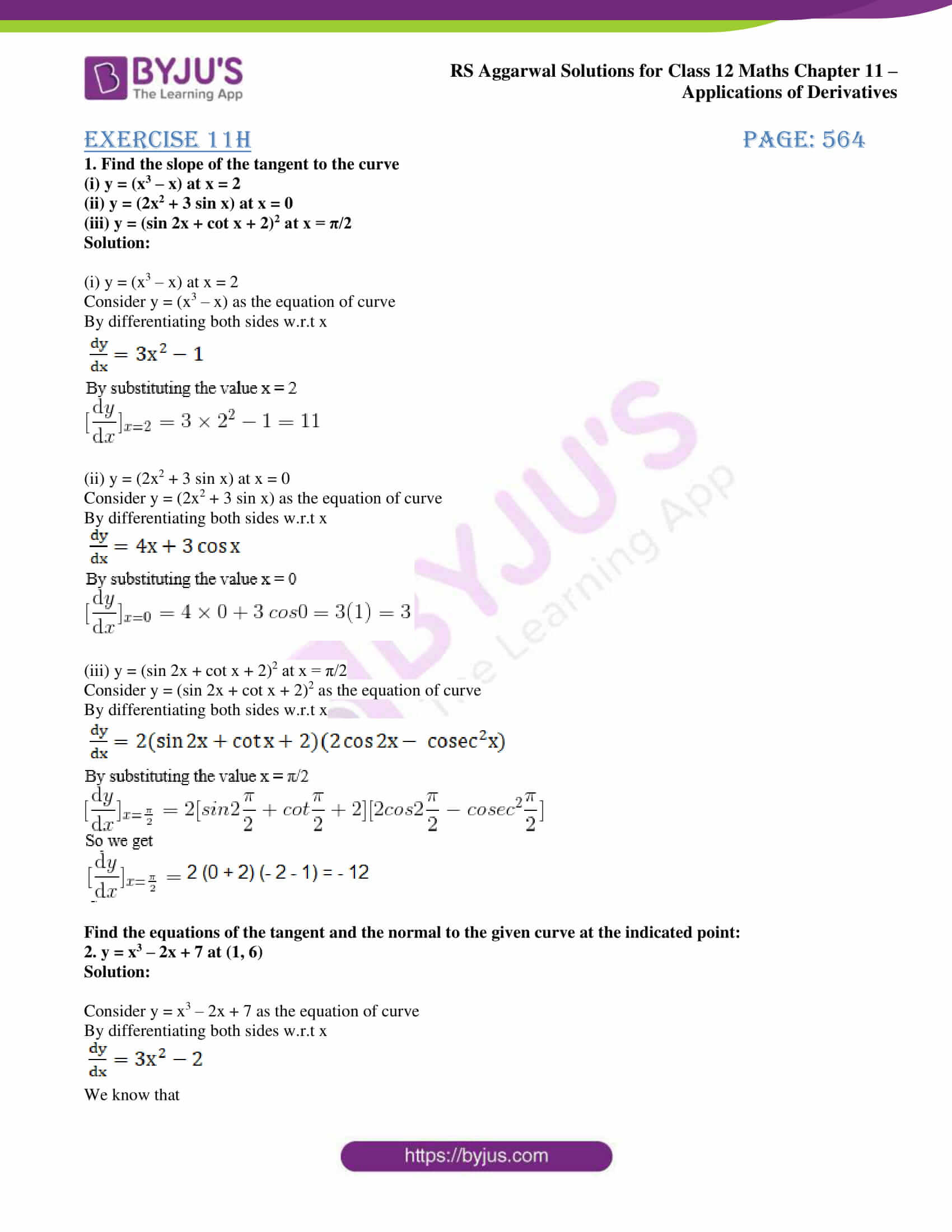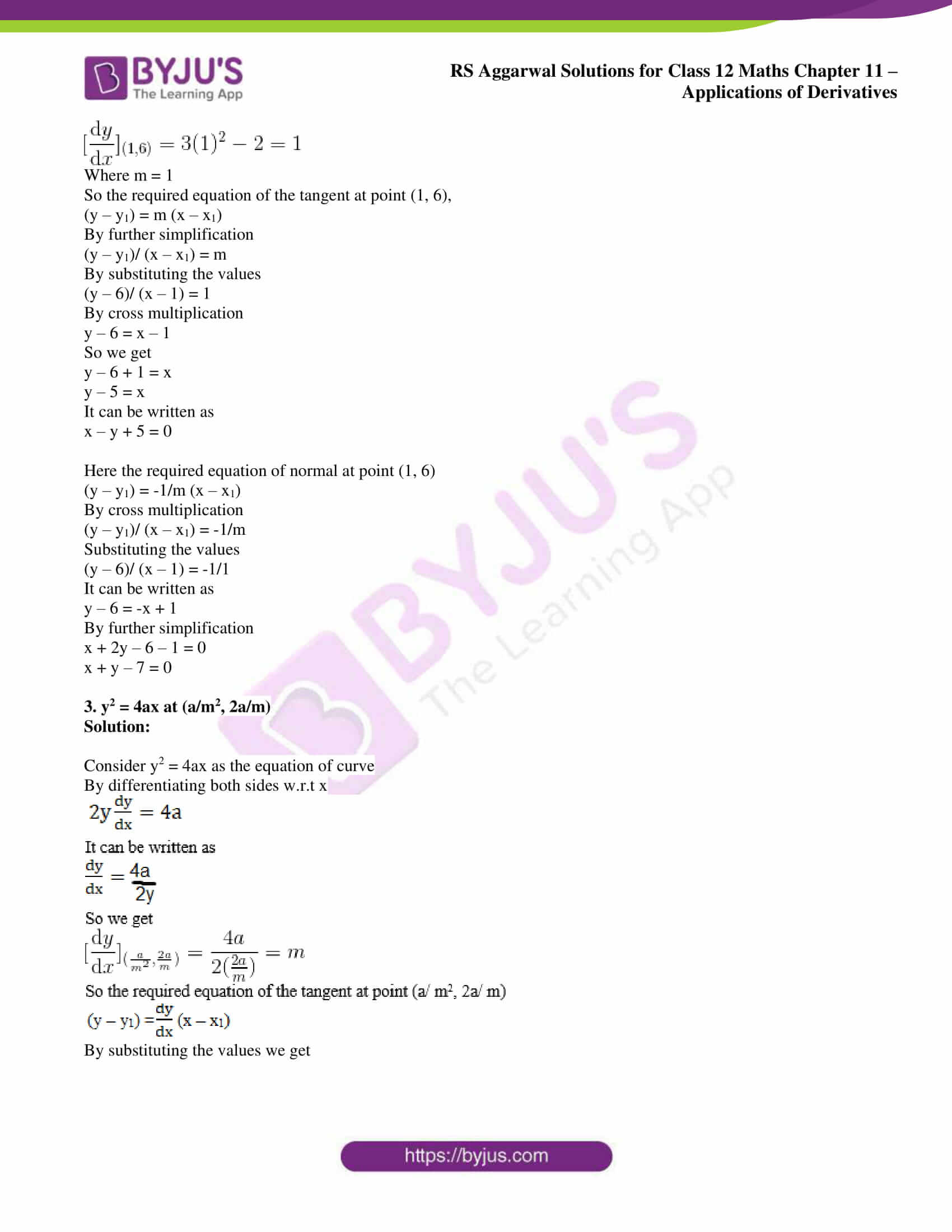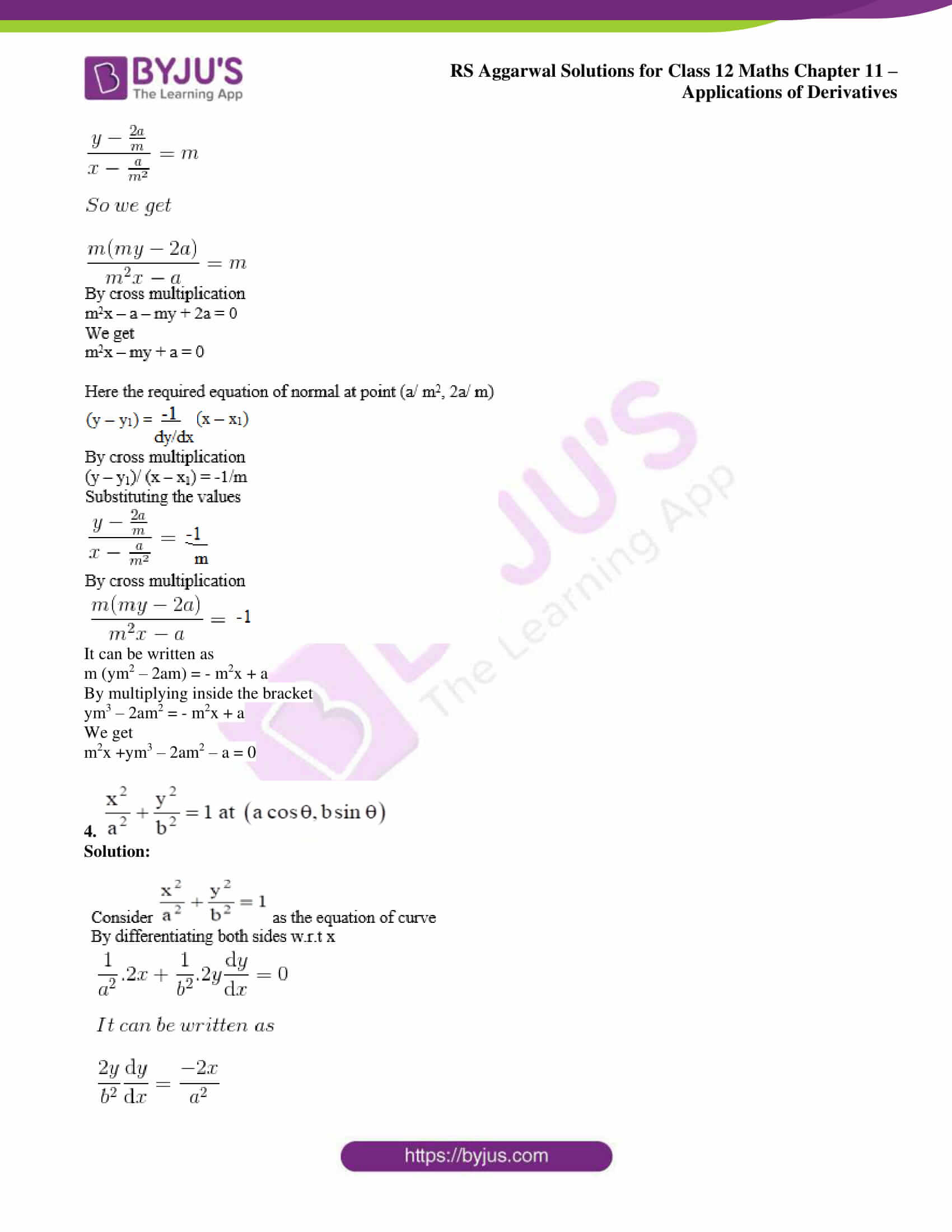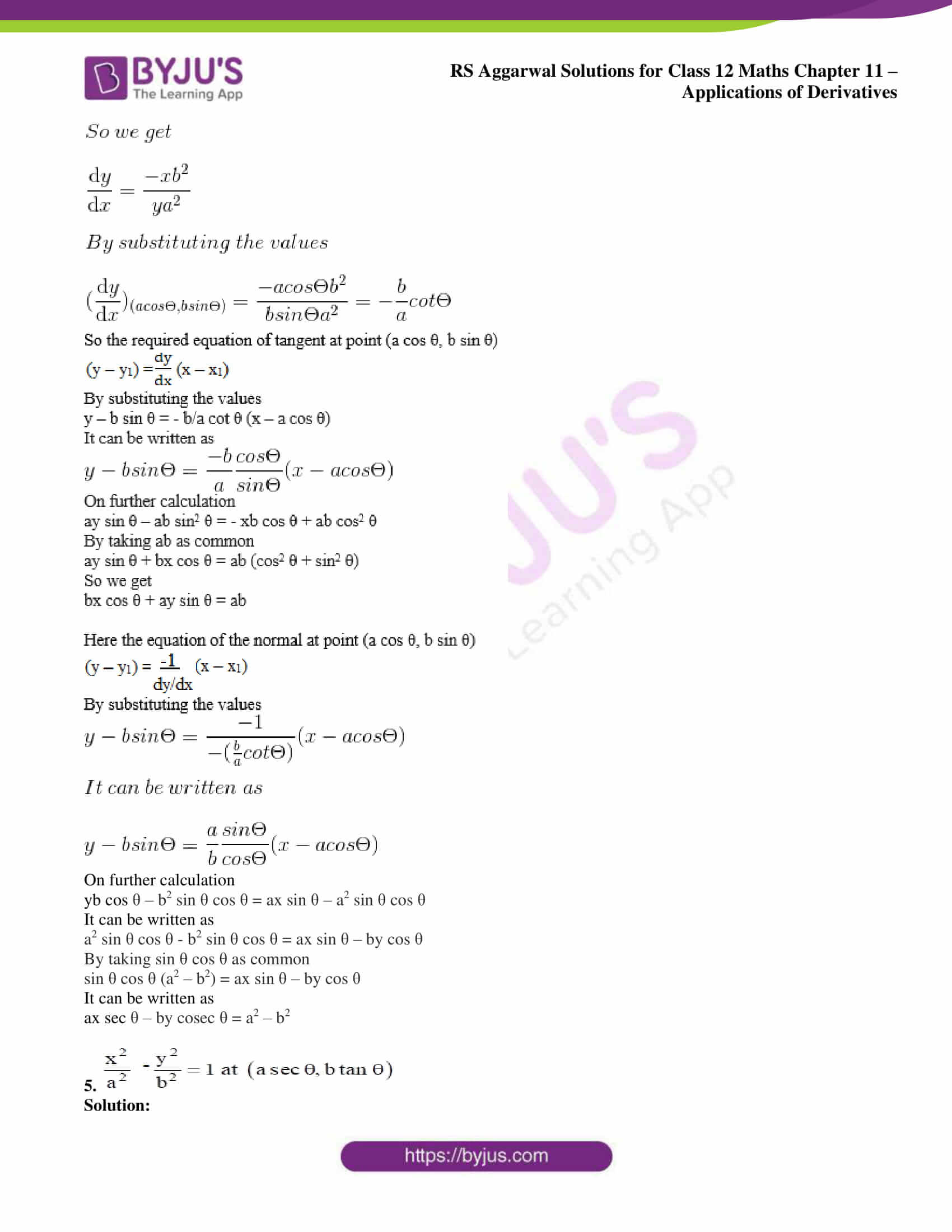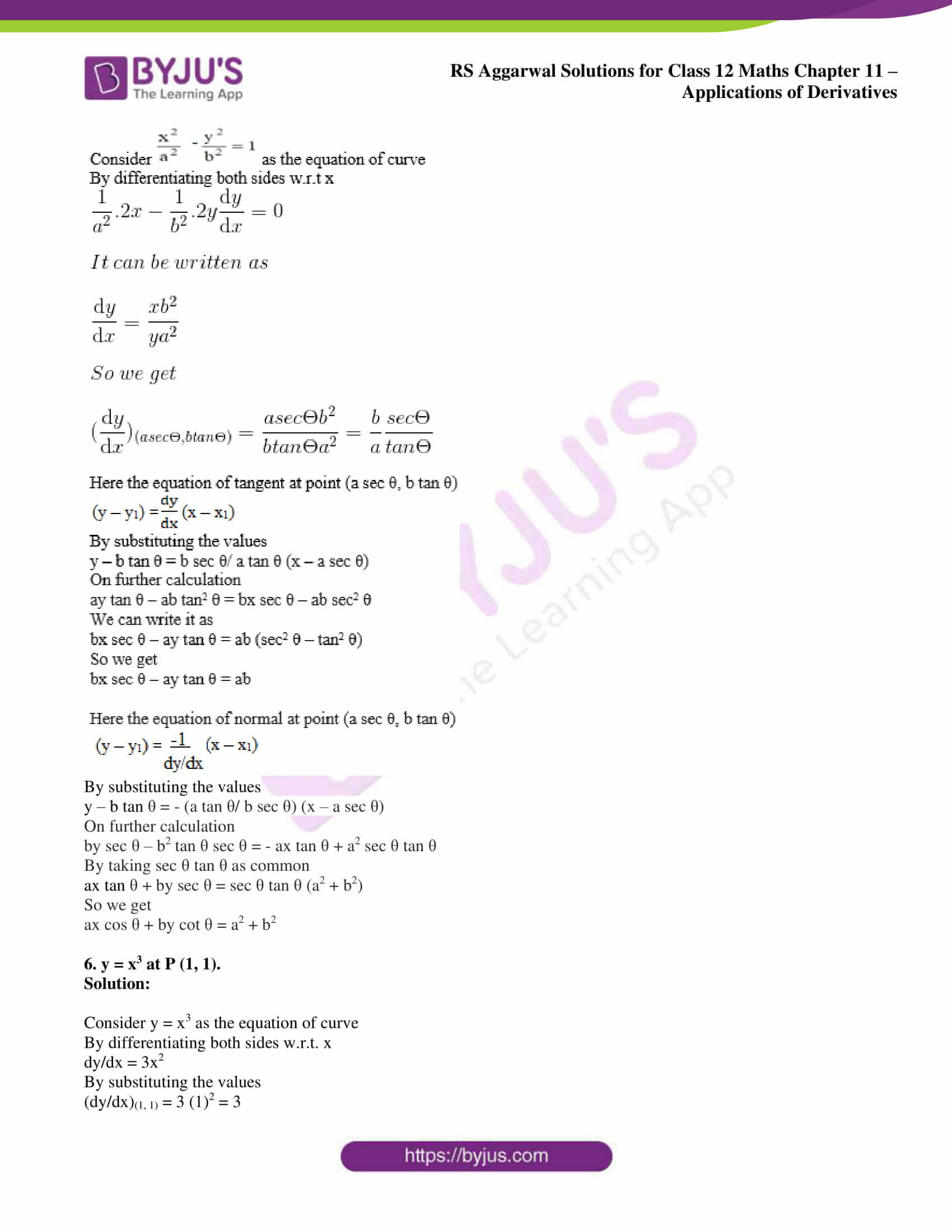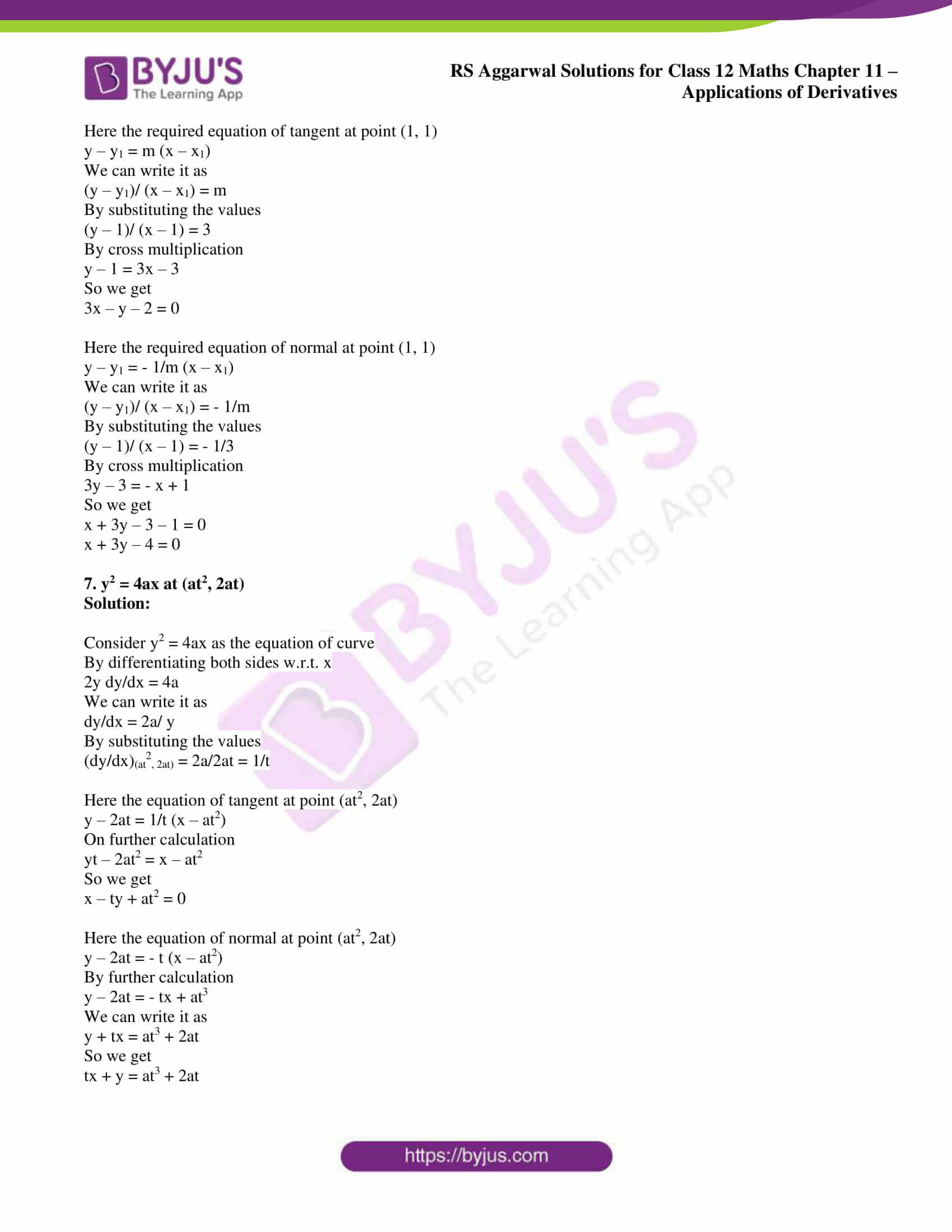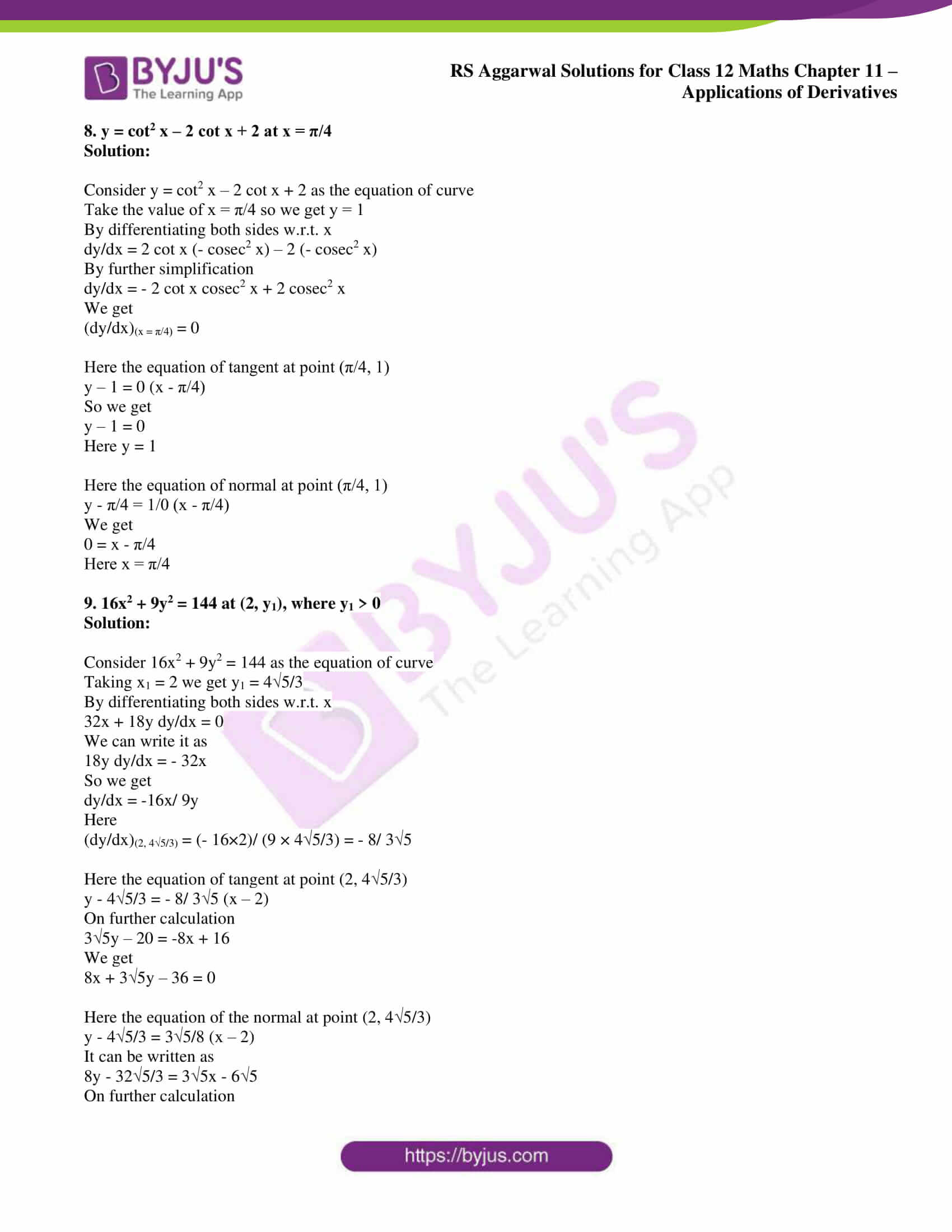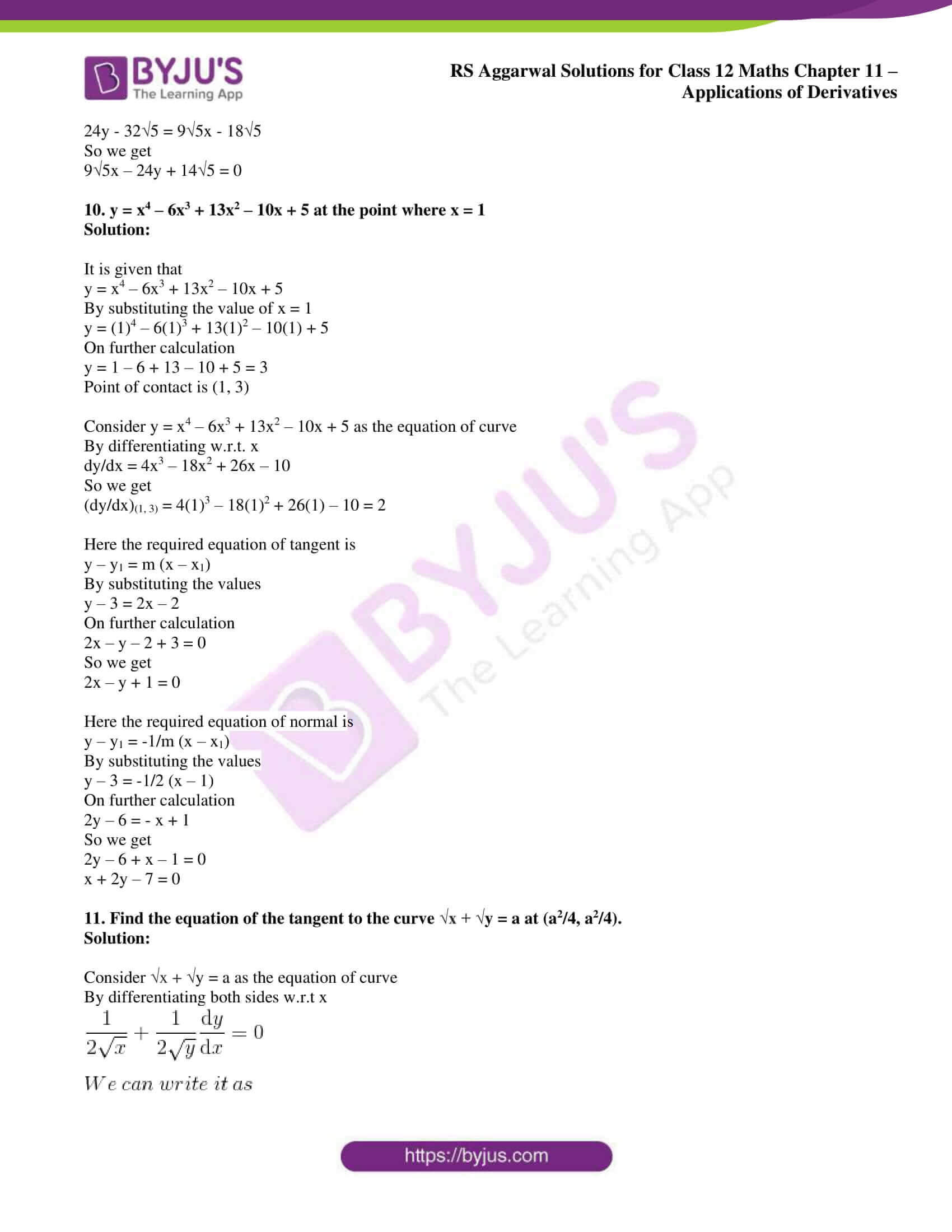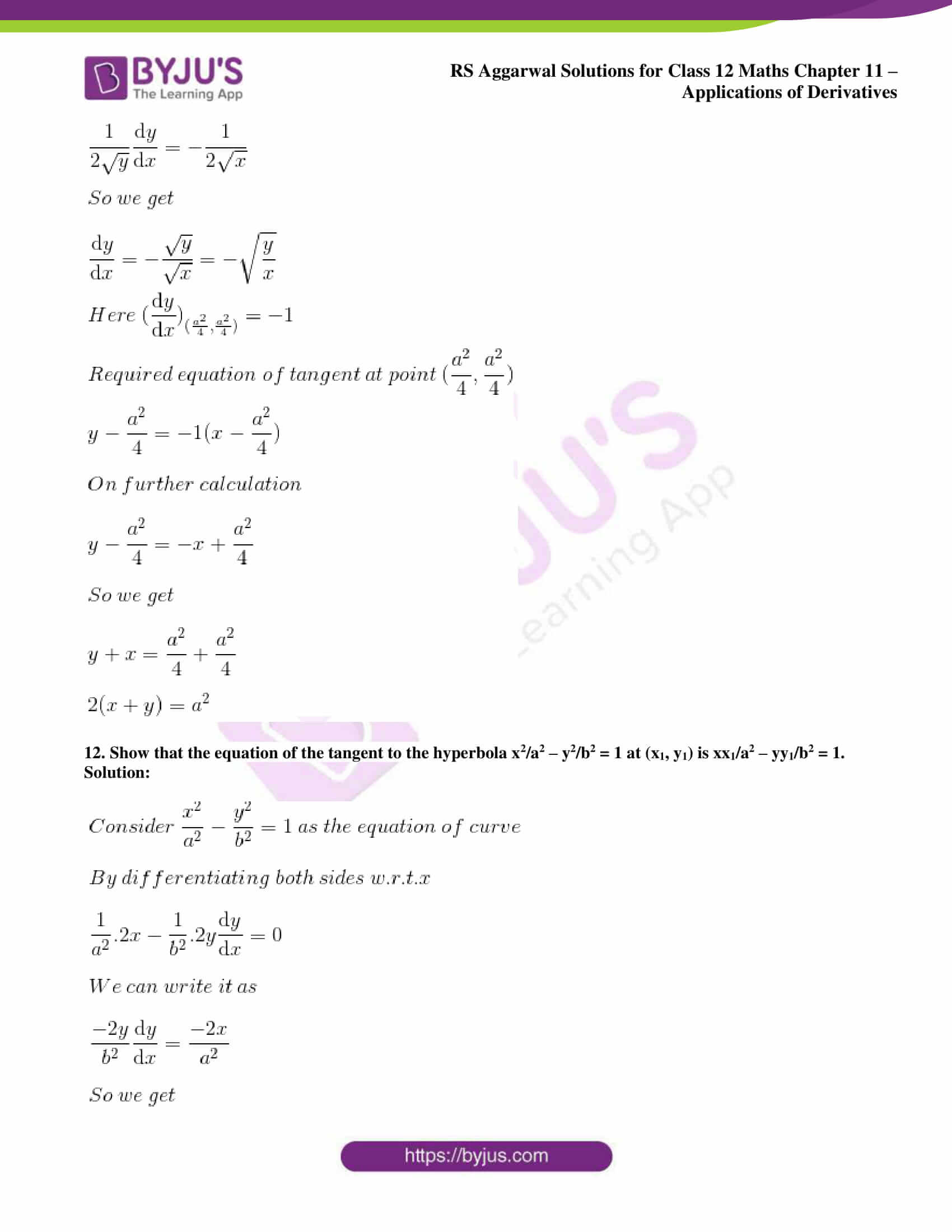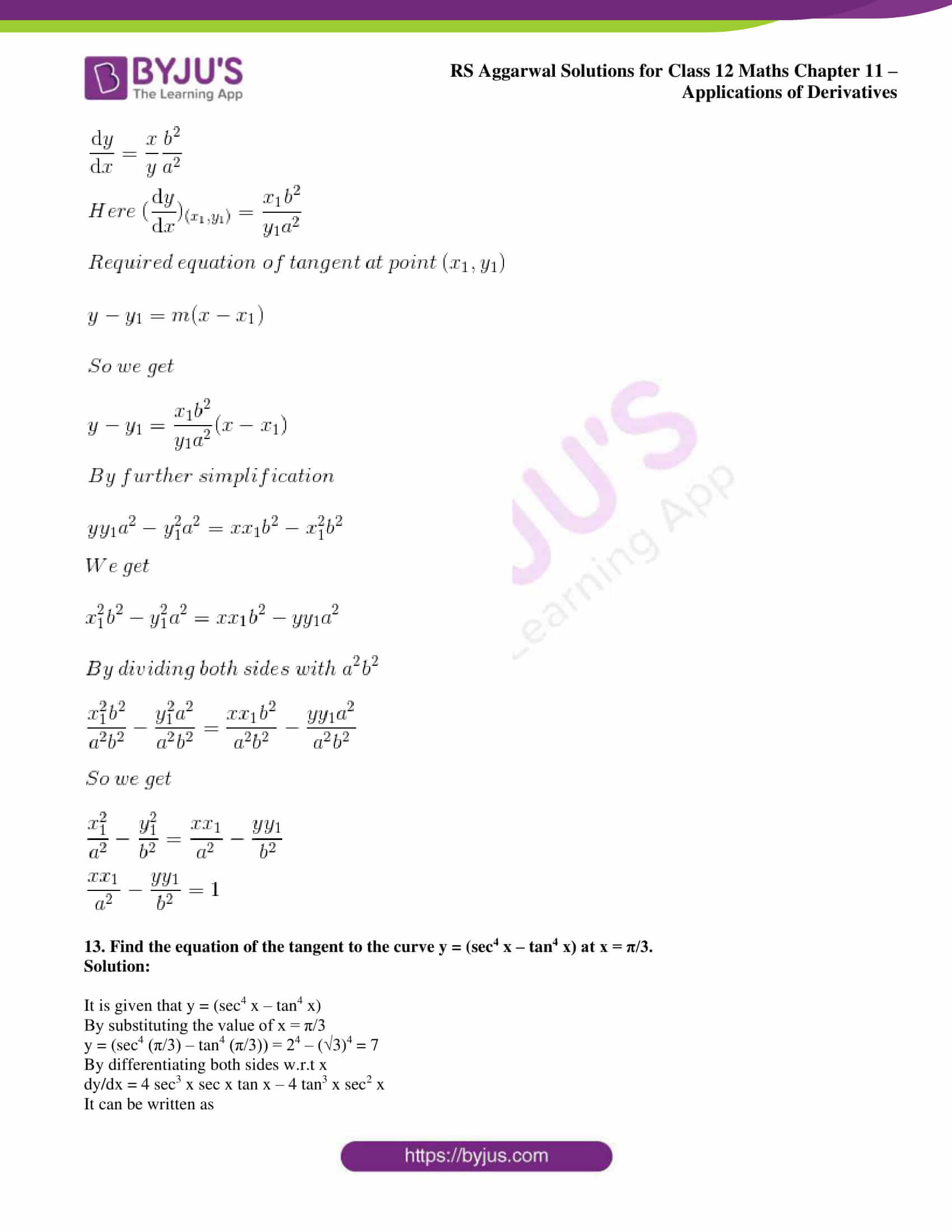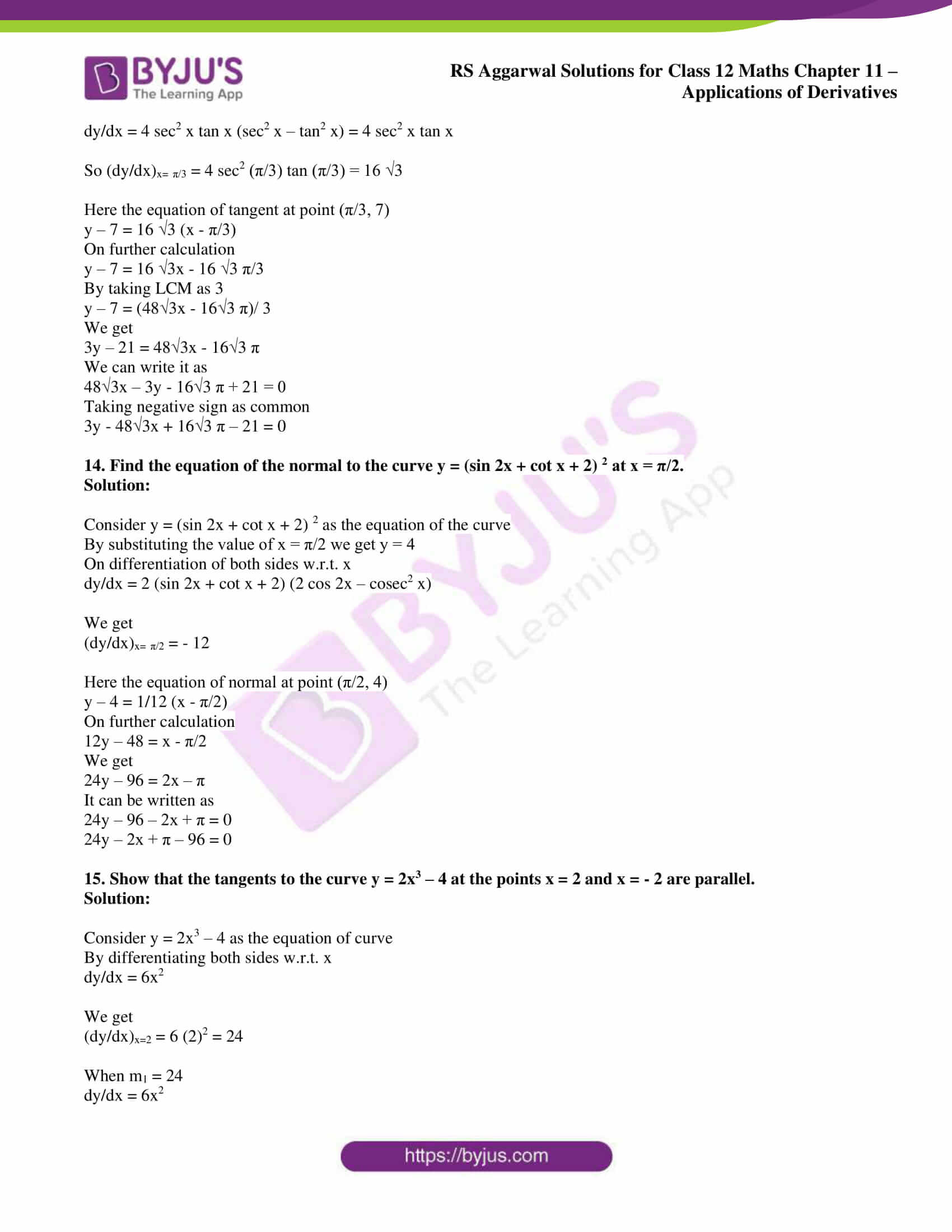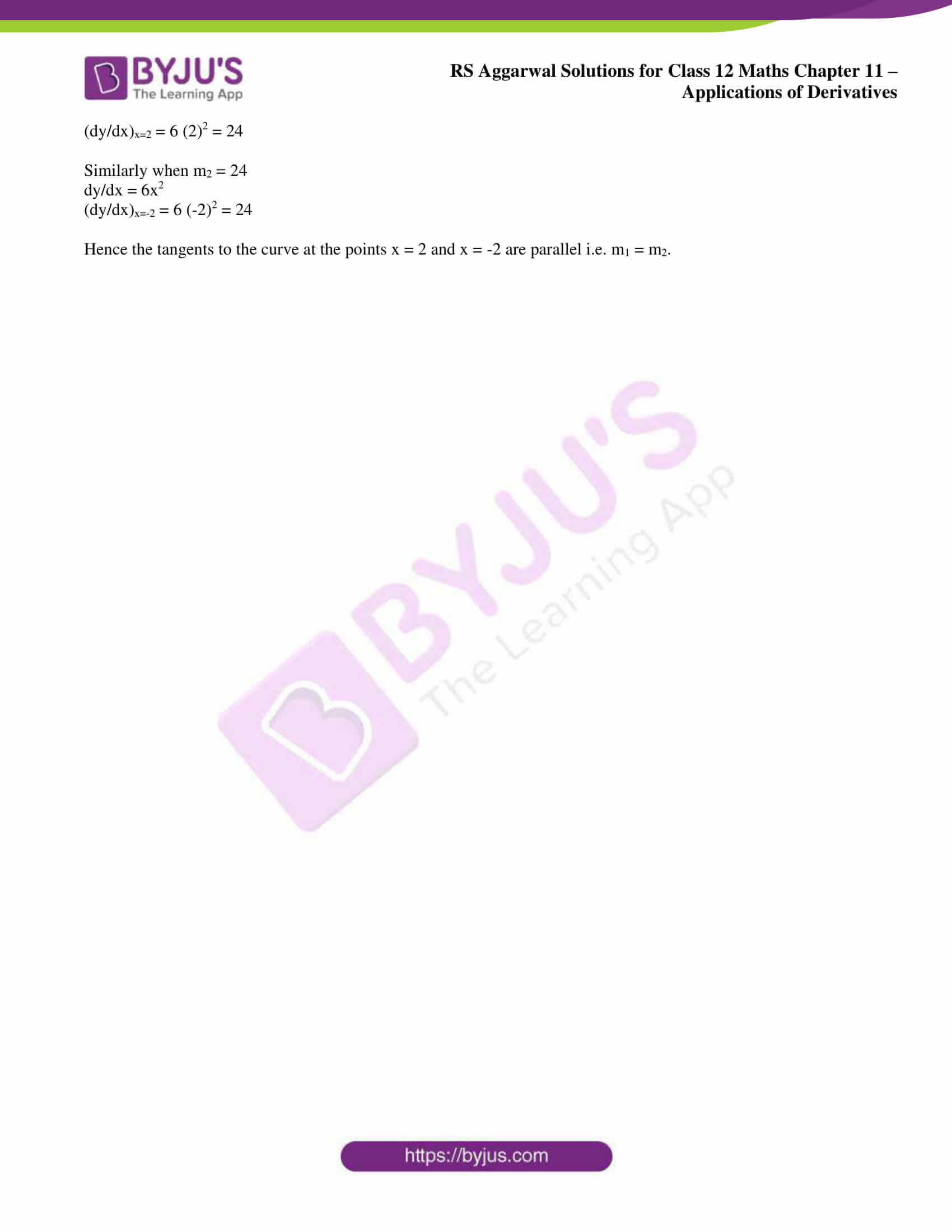### Access other exercise solutions of Class 12 Maths Chapter 11: Applications of Derivatives

Exercise 11A Solutions

Exercise 11B Solutions

Exercise 11C Solutions

Exercise 11D Solutions

Exercise 11E Solutions

Exercise 11F Solutions

Exercise 11G Solutions

### Access RS Aggarwal Solutions for Class 12 Maths Chapter 11: Applications of Derivatives Exercise 11H

1. Find the slope of the tangent to the curve

(i) y = (x3 – x) at x = 2

(ii) y = (2x2 + 3 sin x) at x = 0

(iii) y = (sin 2x + cot x + 2)2 at x = π/2

Solution:

(i) y = (x3 – x) at x = 2

Consider y = (x3 – x) as the equation of curve

By differentiating both sides w.r.t x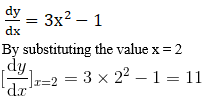(ii) y = (2x2 + 3 sin x) at x = 0

Consider y = (2x2 + 3 sin x) as the equation of curve

By differentiating both sides w.r.t x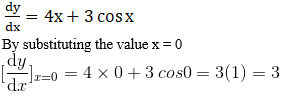(iii) y = (sin 2x + cot x + 2)2 at x = π/2

Consider y = (sin 2x + cot x + 2)2 as the equation of curve

By differentiating both sides w.r.t x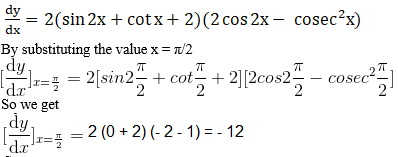Find the equations of the tangent and the normal to the given curve at the indicated point:

2. y = x3 – 2x + 7 at (1, 6)

Solution:

Consider y = x3 – 2x + 7 as the equation of curve

By differentiating both sides w.r.t x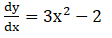We know that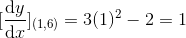Where m = 1

So the required equation of the tangent at point (1, 6),

(y – y1) = m (x – x1)

By further simplification

(y – y1)/ (x – x1) = m

By substituting the values

(y – 6)/ (x – 1) = 1

By cross multiplication

y – 6 = x – 1

So we get

y – 6 + 1 = x

y – 5 = x

It can be written as

x – y + 5 = 0

Here the required equation of normal at point (1, 6)

(y – y1) = -1/m (x – x1)

By cross multiplication

(y – y1)/ (x – x1) = -1/m

Substituting the values

(y – 6)/ (x – 1) = -1/1

It can be written as

y – 6 = -x + 1

By further simplification

x + 2y – 6 – 1 = 0

x + y – 7 = 0

3. y2 = 4ax at (a/m2, 2a/m)

Solution:

Consider y2 = 4ax as the equation of curve

By differentiating both sides w.r.t x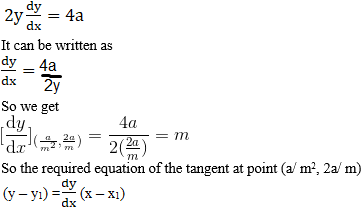By substituting the values we get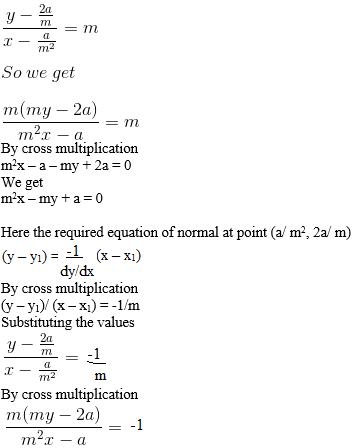It can be written as

m (ym2 – 2am) = – m2x + a

By multiplying inside the bracket

ym3 – 2am2 = – m2x + a

We get

m2x +ym3 – 2am2 – a = 0

4.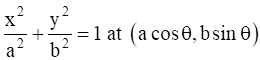Solution: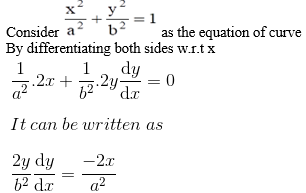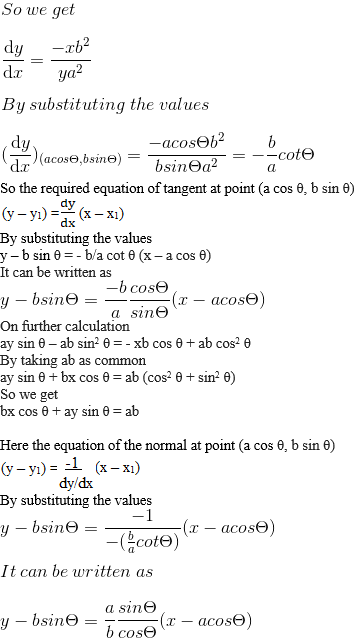On further calculation

yb cos θ – b2 sin θ cos θ = ax sin θ – a2 sin θ cos θ

It can be written as

a2 sin θ cos θ – b2 sin θ cos θ = ax sin θ – by cos θ

By taking sin θ cos θ as common

sin θ cos θ (a2 – b2) = ax sin θ – by cos θ

It can be written as

ax sec θ – by cosec θ = a2 – b2

5.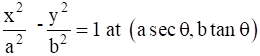Solution: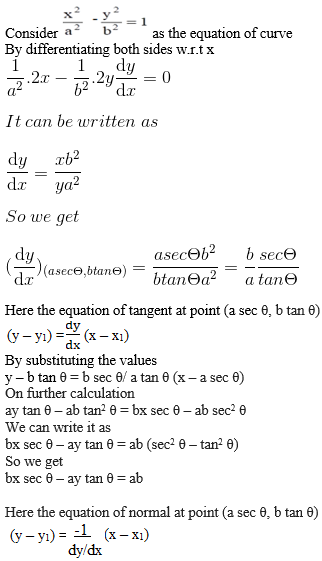By substituting the values

y – b tan θ = – (a tan θ/ b sec θ) (x – a sec θ)

On further calculation

by sec θ – b2 tan θ sec θ = – ax tan θ + a2 sec θ tan θ

By taking sec θ tan θ as common

ax tan θ + by sec θ = sec θ tan θ (a2 + b2)

So we get

ax cos θ + by cot θ = a2 + b2

6. y = x3 at P (1, 1).

Solution:

Consider y = x3 as the equation of curve

By differentiating both sides w.r.t. x

dy/dx = 3x2

By substituting the values

(dy/dx)(1, 1) = 3 (1)2 = 3

Here the required equation of tangent at point (1, 1)

y – y1 = m (x – x1)

We can write it as

(y – y1)/ (x – x1) = m

By substituting the values

(y – 1)/ (x – 1) = 3

By cross multiplication

y – 1 = 3x – 3

So we get

3x – y – 2 = 0

Here the required equation of normal at point (1, 1)

y – y1 = – 1/m (x – x1)

We can write it as

(y – y1)/ (x – x1) = – 1/m

By substituting the values

(y – 1)/ (x – 1) = – 1/3

By cross multiplication

3y – 3 = – x + 1

So we get

x + 3y – 3 – 1 = 0

x + 3y – 4 = 0

7. y2 = 4ax at (at2, 2at)

Solution:

Consider y2 = 4ax as the equation of curve

By differentiating both sides w.r.t. x

2y dy/dx = 4a

We can write it as

dy/dx = 2a/ y

By substituting the values

(dy/dx)(at2, 2at) = 2a/2at = 1/t

Here the equation of tangent at point (at2, 2at)

y – 2at = 1/t (x – at2)

On further calculation

yt – 2at2 = x – at2

So we get

x – ty + at2 = 0

Here the equation of normal at point (at2, 2at)

y – 2at = – t (x – at2)

By further calculation

y – 2at = – tx + at3

We can write it as

y + tx = at3 + 2at

So we get

tx + y = at3 + 2at

8. y = cot2 x – 2 cot x + 2 at x = π/4

Solution:

Consider y = cot2 x – 2 cot x + 2 as the equation of curve

Take the value of x = π/4 so we get y = 1

By differentiating both sides w.r.t. x

dy/dx = 2 cot x (- cosec2 x) – 2 (- cosec2 x)

By further simplification

dy/dx = – 2 cot x cosec2 x + 2 cosec2 x

We get

(dy/dx)(x = π/4) = 0

Here the equation of tangent at point (π/4, 1)

y – 1 = 0 (x – π/4)

So we get

y – 1 = 0

Here y = 1

Here the equation of normal at point (π/4, 1)

y – π/4 = 1/0 (x – π/4)

We get

0 = x – π/4

Here x = π/4

9. 16x2 + 9y2 = 144 at (2, y1), where y1 > 0

Solution:

Consider 16x2 + 9y2 = 144 as the equation of curve

Taking x1 = 2 we get y1 = 45/3

By differentiating both sides w.r.t. x

32x + 18y dy/dx = 0

We can write it as

18y dy/dx = – 32x

So we get

dy/dx = -16x/ 9y

Here

(dy/dx)(2, 45/3) = (- 16×2)/ (9 × 45/3) = – 8/ 35

Here the equation of tangent at point (2, 45/3)

y – 45/3 = – 8/ 35 (x – 2)

On further calculation

35y – 20 = -8x + 16

We get

8x + 35y – 36 = 0

Here the equation of the normal at point (2, 45/3)

y – 45/3 = 35/8 (x – 2)

It can be written as

8y – 325/3 = 35x – 65

On further calculation

24y – 325 = 95x – 185

So we get

95x – 24y + 145 = 0

10. y = x4 – 6x3 + 13x2 – 10x + 5 at the point where x = 1

Solution:

It is given that

y = x4 – 6x3 + 13x2 – 10x + 5

By substituting the value of x = 1

y = (1)4 – 6(1)3 + 13(1)2 – 10(1) + 5

On further calculation

y = 1 – 6 + 13 – 10 + 5 = 3

Point of contact is (1, 3)

Consider y = x4 – 6x3 + 13x2 – 10x + 5 as the equation of curve

By differentiating w.r.t. x

dy/dx = 4x3 – 18x2 + 26x – 10

So we get

(dy/dx)(1, 3) = 4(1)3 – 18(1)2 + 26(1) – 10 = 2

Here the required equation of tangent is

y – y1 = m (x – x1)

By substituting the values

y – 3 = 2x – 2

On further calculation

2x – y – 2 + 3 = 0

So we get

2x – y + 1 = 0

Here the required equation of normal is

y – y1 = -1/m (x – x1)

By substituting the values

y – 3 = -1/2 (x – 1)

On further calculation

2y – 6 = – x + 1

So we get

2y – 6 + x – 1 = 0

x + 2y – 7 = 0

11. Find the equation of the tangent to the curve √x + √y = a at (a2/4, a2/4).

Solution:

Consider √x + √y = a as the equation of curve

By differentiating both sides w.r.t x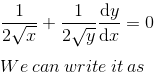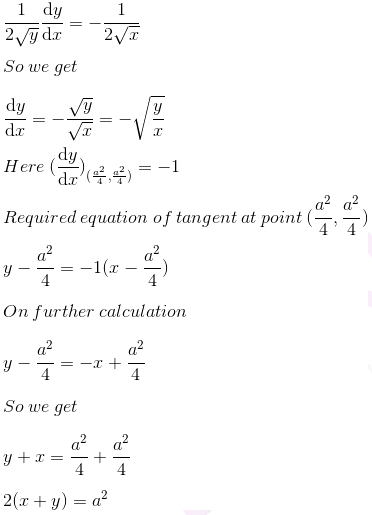12. Show that the equation of the tangent to the hyperbola x2/a2 – y2/b2 = 1 at (x1, y1) is xx1/a2 – yy1/b2 = 1.

Solution: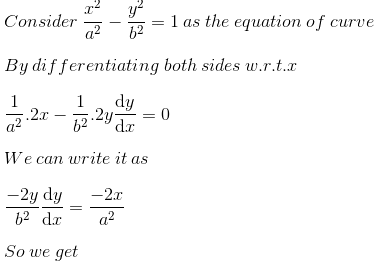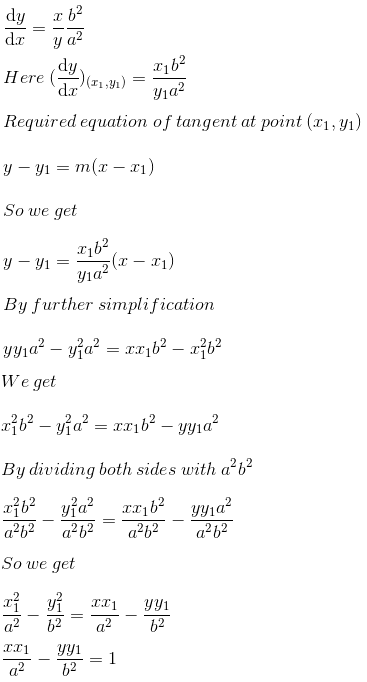13. Find the equation of the tangent to the curve y = (sec4 x – tan4 x) at x = π/3.

Solution:

It is given that y = (sec4 x – tan4 x)

By substituting the value of x = π/3

y = (sec4 (π/3) – tan4 (π/3)) = 24 – (√3)4 = 7

By differentiating both sides w.r.t x

dy/dx = 4 sec3 x sec x tan x – 4 tan3 x sec2 x

It can be written as

dy/dx = 4 sec2 x tan x (sec2 x – tan2 x) = 4 sec2 x tan x

So (dy/dx)x= π/3 = 4 sec2 (π/3) tan (π/3) = 16 √3

Here the equation of tangent at point (π/3, 7)

y – 7 = 16 √3 (x – π/3)

On further calculation

y – 7 = 16 √3x – 16 √3 π/3

By taking LCM as 3

y – 7 = (48√3x – 16√3 π)/ 3

We get

3y – 21 = 48√3x – 16√3 π

We can write it as

48√3x – 3y – 16√3 π + 21 = 0

Taking negative sign as common

3y – 48√3x + 16√3 π – 21 = 0

14. Find the equation of the normal to the curve y = (sin 2x + cot x + 2) 2 at x = π/2.

Solution:

Consider y = (sin 2x + cot x + 2) 2 as the equation of the curve

By substituting the value of x = π/2 we get y = 4

On differentiation of both sides w.r.t. x

dy/dx = 2 (sin 2x + cot x + 2) (2 cos 2x – cosec2 x)

We get

(dy/dx)x= π/2 = – 12

Here the equation of normal at point (π/2, 4)

y – 4 = 1/12 (x – π/2)

On further calculation

12y – 48 = x – π/2

We get

24y – 96 = 2x – π

It can be written as

24y – 96 – 2x + π = 0

24y – 2x + π – 96 = 0

15. Show that the tangents to the curve y = 2x3 – 4 at the points x = 2 and x = – 2 are parallel.

Solution:

Consider y = 2x3 – 4 as the equation of curve

By differentiating both sides w.r.t. x

dy/dx = 6x2

We get

(dy/dx)x=2 = 6 (2)2 = 24

When m1 = 24

dy/dx = 6x2

(dy/dx)x=2 = 6 (2)2 = 24

Similarly when m2 = 24

dy/dx = 6x2

(dy/dx)x=-2 = 6 (-2)2 = 24

Hence the tangents to the curve at the points x = 2 and x = -2 are parallel i.e. m1 = m2.